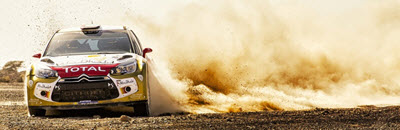# AccelerationAcceleration is how fast velocity changes:

• Speeding up
• Slowing down (also called deceleration)
• Changing direction
• etc

It is usually shown as:

m/s2
"meters per second squared"

What is this "per second squared" thing? An example will help:### A runner accelerates from 5 m/s (5 meters per second) to 6 m/sin just one second

So they accelerate by 1 meter per second per second

See how "per second" is used twice?

It can be thought of as (m/s)/s but is usually written m/s2

So their acceleration is 1 m/s2

The formula is:

Acceleration = Change in Velocity (m/s) Time (s)

### Example: A bike race!

You are cruising along in a bike race, going a steady 10 meters per second (10 m/s).Acceleration: Now you start cycling faster! You increase to 14 m/s over the next 2 seconds (still heading in the same direction):Your velocity increases by 4 m/s, over a time period of 2 seconds, so:

Acceleration = Change in Velocity (m/s) Time (s)

= 4 m/s 2 s = 2 m/s2

Your speed changes by 2 meters per second per second.

### Example: You are running at 7 m/s, and skid to a halt in 2 seconds.

You went from 7 m/s to 0, so that is a decrease in speed:

Acceleration = Change in Velocity (m/s) Time (s)

= −7 m/s 2 s = −3.5 m/s2

We don't always say it, but acceleration has direction (making it a vector):

### A car is heading West at 16 m/s.The driver flicks the wheel, and within 4 seconds has the car headed East at 16 m/s. What is the acceleration?

The numbers are the same, but the direction is different!

Acceleration = Change in Velocity (m/s) Time (s)

Acceleration = From 16 m/s West to 16 m/s East 4 s

From 16 m/s West to 16 m/s East is a total change of 32 m/s towards the East.

Acceleration = 32 m/s East 4 s = 8 m/s2 EastFor more complicated direction changes read vectors.